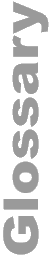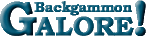Kleinman Doubling FormulaKleinman Doubling Formula [Proposed by Danny Kleinman.]  A guideline for cube handling in pure race positions. Compute K = (D+4)*(D+4) / (S-4), where D is the player's pip count minus the opponent's count, and S is the sum of the pip counts. Kleinman says a player should make an initial double if K > 0.44, or redouble if K > 0.61, and the opponent should accept a double or redouble if K < 1.2. See post by: Øystein Johansen.

 Help   •   About the Glossary   •   List of Terms   •   Full Glossary